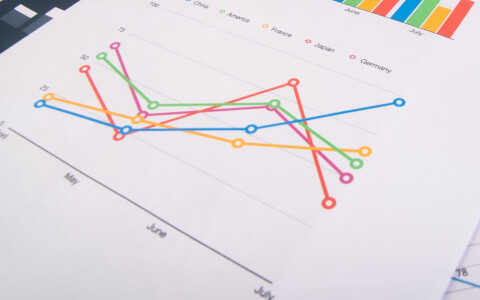# Microsoft Excel Resources9 min

## Variance Formula Excel

Variance is a number that gives a general idea of the dispersion of a dataset. Learn about the variance formula in Excel.12 min

## How to Merge Cells and Split Cells in Excel

Excel has several features which give us the ability to merge and split cells in multiple ways. Here’s how to merge cells in Excel and split cells ...11 min

## How to Make a Line Graph in Excel

Line graphs are some of the most common types of charts used to display continuous data. Here’s how to make a line graph in Excel.12 min

## How to Use the SUM Function in Excel

One of the best functions for beginners to learn is the SUM function in Excel. Start learning with this comprehensive tutorial.10 min

## How to Filter in Excel

Have you ever wanted to temporarily display only certain data from a larger dataset without deleting the rest? Learn how to filter in Excel.10 min

## LEN Function Excel - A Beginner's Guide

The LEN Excel function returns the number of characters in a text string. Learn all about it in this beginner's guide.3 min

## Excel Challenge # 6

It’s coming up to the end of the year and there’s a scramble to take vacation days. Can you help your HR department solve this challenge?10 min

## How to Calculate Percentage in Excel

If you do lots of work with numbers in Excel, then you’ll need to calculate percentages quite often. Learn how to calculate percentages in Excel.

## Join 302,098 serial learners12 min

## How to Create a Drop Down List in Excel

Excel drop down lists are an easy way to control the values entered in a cell. We’ll explore how to create a drop down list in Excel using three m...9 min

## How to Use the IFERROR Excel Function

The IFERROR Excel function is a logical function that tells Excel what to do if the formula that was entered returns an error response. Learn how t...8 min

## How to Multiply in Excel

There are two main ways to do multiplication in Excel. Learn how to multiply in Excel here.8 min

## How to Insert Excel Checkboxes

Did you know you can use Excel to insert checkboxes for to-do lists, dynamic charts, and dashboards? Learn about Excel checkboxes in this beginner'...8 min

## How to Make a Bar Graph in Excel

Bar graphs are an effective way to visually compare frequency, amounts, or units. Learn how to make a bar graph in Excel.8 min

## PMT Function Excel

Learn how to use the Excel PMT function in this comprehensive beginner's guide.10 min

## How to Concatenate in Excel

There are four ways to concatenate in Excel (the CONCATENATE Excel function, CONCAT, TEXTJOIN, and the & operator). Learn about them in this beginn...7 min

## How to Insert a Check Mark in Excel

In this tutorial, we’ll show you how to insert a check mark in Excel. If you use Excel to generate and execute lists check marks will come in handy.4 min

## How to Use the Excel COUNTA Function

The Excel COUNTA function counts all cells with data. Learn all about it in this beginner's guide.8 min

## How to Use the COUNT Function in Excel

Excel is all about large quantities of data. Learn how to use the COUNT function in Excel to count your data in various ways.10 min

## What is INDEX MATCH & Why Should You Use It?

INDEX MATCH is a great way to overcome the limitations of VLOOKUP. In this resource we explore how and when to use it.8 min

## How to Count Unique Values in Excel with the UNIQUE Function

To help you count unique values in Excel, try the UNIQUE function. Learn all about it in this beginner's guide.